# Magnetism

Magnetism

Magnetism is a property of materials that respond at an atomic or subatomic level to an applied magnetic field. Ferromagnetism is the strongest and most familiar type of magnetism. It is responsible for the behavior of permanent magnets, which produce their own persistent magnetic fields, as well as the materials that are attracted to them. However, all materials are influenced to a greater or lesser degree by the presence of a magnetic field. Some are attracted to a magnetic field (paramagnetism); others are repulsed by a magnetic field (diamagnetism); others have a much more complex relationship with an applied magnetic field (spin glass behavior and antiferromagnetism). Substances that are negligibly affected by magnetic fields are known as non-magnetic substances. They include copper, aluminium, gases, and plastic.

The magnetic state (or phase) of a material depends on temperature (and other variables such as pressure and applied magnetic field) so that a material may exhibit more than one form of magnetism depending on its temperature, etc.

## History

Aristotle attributed the first of what could be called a scientific discussion on magnetism to Thales, who lived from about 625 BCE to about 545 BCE. Around the same time, in ancient India, the Indian surgeon, Sushruta, was the first to make use of the magnet for surgical purposes.

In ancient China, the earliest literary reference to magnetism lies in a 4th century BCE book called Book of the Devil Valley Master (鬼谷子): "The lodestone makes iron come or it attracts it." The earliest mention of the attraction of a needle appears in a work composed between AD 20 and 100 (Louen-heng): "A lodestone attracts a needle." The ancient Chinese scientist Shen Kuo (1031–1095) was the first person to write of the magnetic needle compass and that it improved the accuracy of navigation by employing the astronomical concept of true north (Dream Pool Essays, AD 1088), and by the 12th century the Chinese were known to use the lodestone compass for navigation. They sculpted a directional spoon from lodestone in such a way that the handle of the spoon always pointed south.

Alexander Neckham, by 1187, was the first in Europe to describe the compass and its use for navigation. In 1269, Peter Peregrinus de Maricourt wrote the Epistola de magnete, the first extant treatise describing the properties of magnets. In 1282, the properties of magnets and the dry compass were discussed by Al-Ashraf, a Yemeni physicist, astronomer, and geographer.

In 1600, William Gilbert published his De Magnete, Magneticisque Corporibus, et de Magno Magnete Tellure (On the Magnet and Magnetic Bodies, and on the Great Magnet the Earth). In this work he describes many of his experiments with his model earth called the terrella. From his experiments, he concluded that the Earth was itself magnetic and that this was the reason compasses pointed north (previously, some believed that it was the pole star (Polaris) or a large magnetic island on the north pole that attracted the compass).

An understanding of the relationship between electricity and magnetism began in 1819 with work by Hans Christian Oersted, a professor at the University of Copenhagen, who discovered more or less by accident that an electric current could influence a compass needle. This landmark experiment is known as Oersted's Experiment. Several other experiments followed, with André-Marie Ampère, who in 1820 discovered that the magnetic field circulating in a closed-path was related to the current flowing through the perimeter of the path; Carl Friedrich Gauss; Jean-Baptiste Biot and Félix Savart, both of which in 1820 came up with the Biot-Savart Law giving an equation for the magnetic field from a current-carrying wire; Michael Faraday, who in 1831 found that a time-varying magnetic flux through a loop of wire induced a voltage, and others finding further links between magnetism and electricity. James Clerk Maxwell synthesized and expanded these insights into Maxwell's equations, unifying electricity, magnetism, and optics into the field of electromagnetism. In 1905, Einstein used these laws in motivating his theory of special relativity, requiring that the laws held true in all inertial reference frames.

Electromagnetism has continued to develop into the 21st century, being incorporated into the more fundamental theories of gauge theory, quantum electrodynamics, electroweak theory, and finally the standard model.

## Sources of magnetism

Magnetism, at its root, arises from two sources:

1. Electric currents or more generally, moving electric charges create magnetic fields (see Maxwell's Equations).
2. Many particles have nonzero "intrinsic" (or "spin") magnetic moments. Just as each particle, by its nature, has a certain mass and charge, each has a certain magnetic moment, possibly zero.

In magnetic materials, sources of magnetization are the electrons' orbital angular motion around the nucleus, and the electrons' intrinsic magnetic moment (see electron magnetic dipole moment). The other sources of magnetism are the nuclear magnetic moments of the nuclei in the material which are typically thousands of times smaller than the electrons' magnetic moments, so they are negligible in the context of the magnetization of materials. Nuclear magnetic moments are important in other contexts, particularly in nuclear magnetic resonance (NMR) and magnetic resonance imaging (MRI).

Ordinarily, the enormous number of electrons in a material are arranged such that their magnetic moments (both orbital and intrinsic) cancel out. This is due, to some extent, to electrons combining into pairs with opposite intrinsic magnetic moments as a result of the Pauli exclusion principle (see electron configuration), or combining into filled subshells with zero net orbital motion. In both cases, the electron arrangement is so as to exactly cancel the magnetic moments from each electron. Moreover, even when the electron configuration is such that there are unpaired electrons and/or non-filled subshells, it is often the case that the various electrons in the solid will contribute magnetic moments that point in different, random directions, so that the material will not be magnetic.

However, sometimes — either spontaneously, or owing to an applied external magnetic field — each of the electron magnetic moments will be, on average, lined up. Then the material can produce a net total magnetic field, which can potentially be quite strong.

The magnetic behavior of a material depends on its structure, particularly its electron configuration, for the reasons mentioned above, and also on the temperature. At high temperatures, random thermal motion makes it more difficult for the electrons to maintain alignment.

## Topics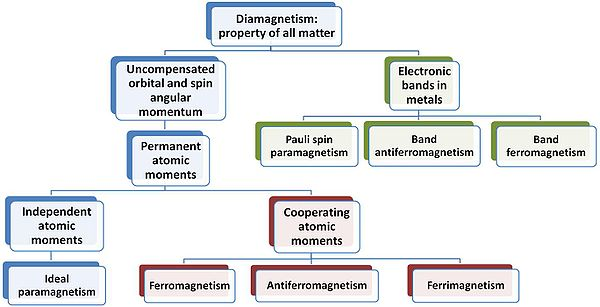Hierarchy of types of magnetism.

### Diamagnetism

Diamagnetism appears in all materials, and is the tendency of a material to oppose an applied magnetic field, and therefore, to be repelled by a magnetic field. However, in a material with paramagnetic properties (that is, with a tendency to enhance an external magnetic field), the paramagnetic behavior dominates. Thus, despite its universal occurrence, diamagnetic behavior is observed only in a purely diamagnetic material. In a diamagnetic material, there are no unpaired electrons, so the intrinsic electron magnetic moments cannot produce any bulk effect. In these cases, the magnetization arises from the electrons' orbital motions, which can be understood classically as follows:

When a material is put in a magnetic field, the electrons circling the nucleus will experience, in addition to their Coulomb attraction to the nucleus, a Lorentz force from the magnetic field. Depending on which direction the electron is orbiting, this force may increase the centripetal force on the electrons, pulling them in towards the nucleus, or it may decrease the force, pulling them away from the nucleus. This effect systematically increases the orbital magnetic moments that were aligned opposite the field, and decreases the ones aligned parallel to the field (in accordance with Lenz's law). This results in a small bulk magnetic moment, with an opposite direction to the applied field.

Note that this description is meant only as an heuristic; a proper understanding requires a quantum-mechanical description.

Note that all materials undergo this orbital response. However, in paramagnetic and ferromagnetic substances, the diamagnetic effect is overwhelmed by the much stronger effects caused by the unpaired electrons.

### Paramagnetism

In a paramagnetic material there are unpaired electrons, i.e. atomic or molecular orbitals with exactly one electron in them. While paired electrons are required by the Pauli exclusion principle to have their intrinsic ('spin') magnetic moments pointing in opposite directions, causing their magnetic fields to cancel out, an unpaired electron is free to align its magnetic moment in any direction. When an external magnetic field is applied, these magnetic moments will tend to align themselves in the same direction as the applied field, thus reinforcing it.

### Ferromagnetism

A ferromagnet, like a paramagnetic substance, has unpaired electrons. However, in addition to the electrons' intrinsic magnetic moment's tendency to be parallel to an applied field, there is also in these materials a tendency for these magnetic moments to orient parallel to each other to maintain a lowered-energy state. Thus, even when the applied field is removed, the electrons in the material maintain a parallel orientation.

Every ferromagnetic substance has its own individual temperature, called the Curie temperature, or Curie point, above which it loses its ferromagnetic properties. This is because the thermal tendency to disorder overwhelms the energy-lowering due to ferromagnetic order.

Some well-known ferromagnetic materials that exhibit easily detectable magnetic properties (to form magnets) are nickel, iron, cobalt, gadolinium and their alloys.

#### Magnetic domains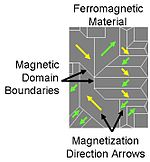Magnetic domains in ferromagnetic material.

The magnetic moment of atoms in a ferromagnetic material cause them to behave something like tiny permanent magnets. They stick together and align themselves into small regions of more or less uniform alignment called magnetic domains or Weiss domains. Magnetic domains can be observed with a magnetic force microscope to reveal magnetic domain boundaries that resemble white lines in the sketch. There are many scientific experiments that can physically show magnetic fields.Effect of a magnet on the domains.

When a domain contains too many molecules, it becomes unstable and divides into two domains aligned in opposite directions so that they stick together more stably as shown at the right.

When exposed to a magnetic field, the domain boundaries move so that the domains aligned with the magnetic field grow and dominate the structure as shown at the left. When the magnetizing field is removed, the domains may not return to an unmagnetized state. This results in the ferromagnetic material's being magnetized, forming a permanent magnet.

When magnetized strongly enough that the prevailing domain overruns all others to result in only one single domain, the material is magnetically saturated. When a magnetized ferromagnetic material is heated to the Curie point temperature, the molecules are agitated to the point that the magnetic domains lose the organization and the magnetic properties they cause cease. When the material is cooled, this domain alignment structure spontaneously returns, in a manner roughly analogous to how a liquid can freeze into a crystalline solid.

### Antiferromagnetism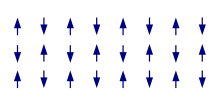Antiferromagnetic ordering

In an antiferromagnet, unlike a ferromagnet, there is a tendency for the intrinsic magnetic moments of neighboring valence electrons to point in opposite directions. When all atoms are arranged in a substance so that each neighbor is 'anti-aligned', the substance is antiferromagnetic. Antiferromagnets have a zero net magnetic moment, meaning no field is produced by them. Antiferromagnets are less common compared to the other types of behaviors, and are mostly observed at low temperatures. In varying temperatures, antiferromagnets can be seen to exhibit diamagnetic and ferrimagnetic properties.

In some materials, neighboring electrons want to point in opposite directions, but there is no geometrical arrangement in which each pair of neighbors is anti-aligned. This is called a spin glass, and is an example of geometrical frustration.

### Ferrimagnetism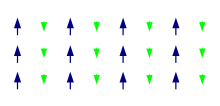Ferrimagnetic ordering

Like ferromagnetism, ferrimagnets retain their magnetization in the absence of a field. However, like antiferromagnets, neighboring pairs of electron spins like to point in opposite directions. These two properties are not contradictory, because in the optimal geometrical arrangement, there is more magnetic moment from the sublattice of electrons that point in one direction, than from the sublattice that points in the opposite direction.

The first discovered magnetic substance, magnetite, was originally believed to be a ferromagnet; Louis Néel disproved this, however, with the discovery of ferrimagnetism.

### Superparamagnetism

When a ferromagnet or ferrimagnet is sufficiently small, it acts like a single magnetic spin that is subject to Brownian motion. Its response to a magnetic field is qualitatively similar to the response of a paramagnet, but much larger.

### Electromagnet

An electromagnet is a type of magnet whose magnetism is produced by the flow of electric current. The magnetic field disappears when the current ceases.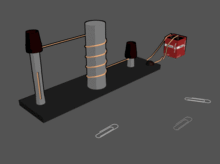Electromagnets attracts paper clips when current is applied creating a magnetic field. The electromagnet loses them when current and magnetic field are removed.

## Magnetism, electricity, and special relativity

As a consequence of Einstein's theory of special relativity, electricity and magnetism are fundamentally interlinked. Both magnetism lacking electricity, and electricity without magnetism, are inconsistent with special relativity, due to such effects as length contraction, time dilation, and the fact that the magnetic force is velocity-dependent. However, when both electricity and magnetism are taken into account, the resulting theory (electromagnetism) is fully consistent with special relativity. In particular, a phenomenon that appears purely electric to one observer may be purely magnetic to another, or more generally the relative contributions of electricity and magnetism are dependent on the frame of reference. Thus, special relativity "mixes" electricity and magnetism into a single, inseparable phenomenon called electromagnetism, analogous to how relativity "mixes" space and time into spacetime.

## Magnetic fields in a material

In a vacuum,$\mathbf{B} \ = \ \mu_0\mathbf{H},$

where μ0 is the vacuum permeability.

In a material,$\mathbf{B} \ = \ \mu_0(\mathbf{H} + \mathbf{M}). \$

The quantity μ0M is called magnetic polarization.

If the field H is small, the response of the magnetization M in a diamagnet or paramagnet is approximately linear:$\mathbf{M} = \chi \mathbf{H},$

the constant of proportionality being called the magnetic susceptibility. If so,$\mu_0(\mathbf{H} + \mathbf{M}) \ = \ \mu_0(1+\chi) \mathbf{H} \ = \ \mu_r\mu_0 \mathbf{H} \ = \ \mu \mathbf{H}.$

In a hard magnet such as a ferromagnet, M is not proportional to the field and is generally nonzero even when H is zero (see Remanence).

## Force due to magnetic field - The magnetic force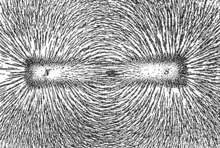Magnetic lines of force of a bar magnet shown by iron filings on paper

The phenomenon of magnetism is "mediated" by the magnetic field. An electric current or magnetic dipole creates a magnetic field, and that field, in turn, imparts magnetic forces on other particles that are in the fields.

Maxwell's equations, which simplify to the Biot-Savart law in the case of steady currents, describe the origin and behavior of the fields that govern these forces. Therefore magnetism is seen whenever electrically charged particles are in motion---for example, from movement of electrons in an electric current, or in certain cases from the orbital motion of electrons around an atom's nucleus. They also arise from "intrinsic" magnetic dipoles arising from quantum-mechanical spin.

The same situations that create magnetic fields — charge moving in a current or in an atom, and intrinsic magnetic dipoles — are also the situations in which a magnetic field has an effect, creating a force. Following is the formula for moving charge; for the forces on an intrinsic dipole, see magnetic dipole.

When a charged particle moves through a magnetic field B, it feels a Lorentz force F given by the cross product:$\mathbf{F} = q (\mathbf{v} \times \mathbf{B})$

where

q is the electric charge of the particle, and
v is the velocity vector of the particle

Because this is a cross product, the force is perpendicular to both the motion of the particle and the magnetic field. It follows that the magnetic force does no work on the particle; it may change the direction of the particle's movement, but it cannot cause it to speed up or slow down. The magnitude of the force is$F=qvB\sin\theta\,$

where θ is the angle between v and B.

One tool for determining the direction of the velocity vector of a moving charge, the magnetic field, and the force exerted is labeling the index finger "V", the middle finger "B", and the thumb "F" with your right hand. When making a gun-like configuration, with the middle finger crossing under the index finger, the fingers represent the velocity vector, magnetic field vector, and force vector, respectively. See also right hand rule.

## Magnetic dipoles

A very common source of magnetic field shown in nature is a dipole, with a "South pole" and a "North pole", terms dating back to the use of magnets as compasses, interacting with the Earth's magnetic field to indicate North and South on the globe. Since opposite ends of magnets are attracted, the north pole of a magnet is attracted to the south pole of another magnet. The Earth's North Magnetic Pole (currently in the Arctic Ocean, north of Canada) is physically a south pole, as it attracts the north pole of a compass.

A magnetic field contains energy, and physical systems move toward configurations with lower energy. When diamagnetic material is placed in a magnetic field, a magnetic dipole tends to align itself in opposed polarity to that field, thereby lowering the net field strength. When ferromagnetic material is placed within a magnetic field, the magnetic dipoles align to the applied field, thus expanding the domain walls of the magnetic domains.

### Magnetic monopoles

Since a bar magnet gets its ferromagnetism from electrons distributed evenly throughout the bar, when a bar magnet is cut in half, each of the resulting pieces is a smaller bar magnet. Even though a magnet is said to have a north pole and a south pole, these two poles cannot be separated from each other. A monopole — if such a thing exists — would be a new and fundamentally different kind of magnetic object. It would act as an isolated north pole, not attached to a south pole, or vice versa. Monopoles would carry "magnetic charge" analogous to electric charge. Despite systematic searches since 1931, as of 2010, they have never been observed, and could very well not exist.

Nevertheless, some theoretical physics models predict the existence of these magnetic monopoles. Paul Dirac observed in 1931 that, because electricity and magnetism show a certain symmetry, just as quantum theory predicts that individual positive or negative electric charges can be observed without the opposing charge, isolated South or North magnetic poles should be observable. Using quantum theory Dirac showed that if magnetic monopoles exist, then one could explain the quantization of electric charge---that is, why the observed elementary particles carry charges that are multiples of the charge of the electron.

Certain grand unified theories predict the existence of monopoles which, unlike elementary particles, are solitons (localized energy packets). The initial results of using these models to estimate the number of monopoles created in the big bang contradicted cosmological observations — the monopoles would have been so plentiful and massive that they would have long since halted the expansion of the universe. However, the idea of inflation (for which this problem served as a partial motivation) was successful in solving this problem, creating models in which monopoles existed but were rare enough to be consistent with current observations.

## Quantum-mechanical origin of magnetism

In principle all kinds of magnetism originate (similar to Superconductivity) from specific quantum-mechanical phenomena which are not easily explained (e.g. Mathematical formulation of quantum mechanics, in particular the chapters on spin and on the Pauli principle). A successful model was developed already in 1927, by Walter Heitler and Fritz London, who derived quantum-mechanically, how hydrogen molecules are formed from hydrogen atoms, i.e. from the atomic hydrogen orbitals uA and uB centered at the nuclei A and B, see below. That this leads to magnetism, is not at all obvious, but will be explained in the following.

According the Heitler-London theory, so-called two-body molecular σ-orbitals are formed, namely the resulting orbital is:$\psi(\mathbf r_1,\,\,\mathbf r_2)=\frac{1}{\sqrt{2}}\,\,\left (u_A(\mathbf r_1)u_B(\mathbf r_2)+u_B(\mathbf r_1)u_A(\mathbf r_2)\right )$

Here the last product means that a first electron, r1, is in an atomic hydrogen-orbital centered at the second nucleus, whereas the second electron runs around the first nucleus. This "exchange" phenomenon is an expression for the quantum-mechanical property that particles with identical properties cannot be distinguished. It is specific not only for the formation of chemical bonds, but as we will see, also for magnetism, i.e. in this connection the term exchange interaction arises, a term which is essential for the origin of magnetism, and which is stronger, roughly by factors 100 and even by 1000, than the energies arising from the electrodynamic dipole-dipole interaction.

As for the spin function χ(s1,s2), which is responsible for the magnetism, we have the already mentioned Pauli's principle, namely that a symmetric orbital (i.e. with the + sign as above) must be multiplied with an antisymmetric spin function (i.e. with a - sign), and vice versa. Thus:$\chi (s_1,\,\,s_2)=\frac{1}{\sqrt{2}}\,\,\left (\alpha (s_1)\beta (s_2)-\beta (s_1)\alpha (s_2)\right )$,

I.e., not only uA and uB must be substituted by α and β, respectively (the first entity means "spin up", the second one "spin down"), but also the sign + by the − sign, and finally ri by the discrete values si (= ±½); thereby we have α( + 1 / 2) = β( − 1 / 2) = 1 and α( − 1 / 2) = β( + 1 / 2) = 0. The "singlet state", i.e. the - sign, means: the spins are antiparallel, i.e. for the solid we have antiferromagnetism, and for two-atomic molecules one has diamagnetism. The tendency to form a (homoeopolar) chemical bond (this means: the formation of a symmetric molecular orbital , i.e. with the + sign) results through the Pauli principle automatically in an antisymmetric spin state (i.e. with the - sign). In contrast, the Coulomb repulsion of the electrons, i.e. the tendency that they try to avoid each other by this repulsion, would lead to an antisymmetric orbital function (i.e. with the - sign) of these two particles, and complementary to a symmetric spin function (i.e. with the + sign, one of the so-called "triplet functions"). Thus, now the spins would be parallel (ferromagnetism in a solid, paramagnetism in two-atomic gases).

The last-mentioned tendency dominates in the metals iron, cobalt and nickel, and in some rare earths, which are ferromagnetic. Most of the other metals, where the first-mentioned tendency dominates, are nonmagnetic (e.g. sodium, aluminium, and magnesium) or antiferromagnetic (e.g. manganese). Diatomic gases are also almost exclusively diamagnetic, and not paramagnetic. However, the oxygen molecule, because of the involvement of π-orbitals, is an exception important for the life-sciences.

The Heitler-London considerations can be generalized to the Heisenberg model of magnetism (Heisenberg 1928).

The explanation of the phenomena is thus essentially based on all subtleties of quantum mechanics, whereas the electrodynamics covers mainly the phenomenology.

## Units of electromagnetism

### SI units related to magnetism

SI electromagnetism units
Symbol Name of Quantity Derived Units Conversion of International to SI base units$\ \Iota$ Electric current ampere (SI base unit)$\mathrm{A=C\ s^{-1}}$$\ Q$ Electric charge coulomb$\mathrm{C=A\ s}$$U,\ \Delta V,\ \Delta\phi,\ \Epsilon$ Potential difference; Electromotive force volt$\mathrm{V=J\ C^{-1}=kg\ A^{-1}m^2s^{-3}}$$R;\ \Zeta;\ \Chi$ Electric resistance; Impedance; Reactance ohm$\mathrm{\Omega=V\ A^{-1}=kg\ m^{2} \ A^{-2}s^{-3}}$$\ \rho$ Resistivity ohm metre$\mathrm{\Omega\ m=kg\ A^{-2}m^3s^{-3}}$$\ \Rho$ Electric power watt$\mathrm{W=V\ A=kg\ m^2s^{-3}}$$\ C$ Capacitance farad$\mathrm{F=C\ V^{-1}=A^2kg^{-1}m^{-2}s^4}$$\mathbf{\Epsilon}$ Electric field strength volt per metre$\mathrm{V\ m^{-1}=C^{-1}N=kg\ A^{-1}m\ s^{-3}}$$\mathbf{D}$ Electric displacement field Coulomb per square metre$\mathrm{C\ m^{-2}=A\ m^{-2}s}$
ε Permittivity farad per metre$\mathrm{F\ m^{-1}=A^{2}kg^{-1}m^{-3}s^{4}}$$\!\chi_e$ Electric susceptibility Dimensionless$\Beta;\ G;\ \Upsilon$ Conductance; Admittance; Susceptance siemens$\ \mathrm{S=\Omega^{-1}=kg^{-1}A^2m^{-2}s^3}$$\gamma,\ \kappa,\ \sigma$ Conductivity siemens per metre$\mathrm{S\ m^{-1}=A^2kg^{-1}m^{-3}s^3}$$\ \mathbf{B}$ Magnetic flux density, Magnetic induction tesla$\mathrm{T=Wb\ m^{-2}=kg\ A^{-1}s^{-2}}$$\ \Phi$ Magnetic flux weber$\mathrm{Wb=V\ s=kg\ A^{-1}m^2s^{-2}}$$\mathbf{H}$ Magnetic field strength ampere per metre$\mathrm{A\ m^{-1}}$$L,\ \Mu$ Inductance henry$\mathrm{H=Wb\ A^{-1}=V\ A^{-1}s=kg\ A^{-2}m^2s^{-2}}$$\ \mu$ Permeability henry per metre$\mathrm{H m^{-1}=kg\ A^{-2}m\ s^{-2}}$$\ \chi$ Magnetic susceptibility Dimensionless

### Other units

• gauss – The gauss, abbreviated as G, is the CGS unit of magnetic field (B).
• oersted – The oersted is the CGS unit of magnetizing field (H).
• Maxwell – is the CGS unit for the magnetic flux.
• gamma – is a unit of magnetic flux density that was commonly used before the tesla became popular (1 gamma = 1 nT)
• μ0 – common symbol for the permeability of free space (4π×10−7 N/(ampere-turn)2).

## Living things

Some organisms can detect magnetic fields, a phenomenon known as magnetoception. Magnetobiology studies magnetic fields as a medical treatment; fields naturally produced by an organism are known as biomagnetism.

Wikimedia Foundation. 2010.

Synonyms:

### Look at other dictionaries:

• magnetism — MAGNETÍSM s.n. 1. Proprietatea pe care o au corpurile magnetice de a se magnetiza. ♢ Magnetism terestru (sau pământesc) = totalitatea fenomenelor magnetice caracteristice Pământului. Magnetism animal = fluid universal care ar străbate toate… …   Dicționar Român

• Magnetism — Mag net*ism, n. [Cf. F. magn[ e]tisme.] The property, quality, or state, of being magnetic; the manifestation of the force in nature which is seen in a magnet. At one time it was believed to be separate from the electrical force, but it is now… …   The Collaborative International Dictionary of English

• magnetism — index draw (attraction) Burton s Legal Thesaurus. William C. Burton. 2006 …   Law dictionary

• magnetism — (n.) 1610s, from Mod.L. magnetismus (see MAGNET (Cf. magnet) + ISM (Cf. ism)). Figurative sense of personal charm is from 1650s; in the hypnotic sense it is from Mesmer (see MESMERIZE (Cf. mesmerize)). Meaning science of magnetics is recorded… …   Etymology dictionary

• magnetism — [n] charm, attractiveness allure, appeal, attraction, captivatingness, charisma, draw, drawing power, enchantment, fascination, glamour, hypnotism, influence, lure, magic, mesmerism, power, pull, seductiveness, spell, witchcraft, witchery;… …   New thesaurus

• magnetism — ► NOUN 1) the property displayed by magnets and produced by the motion of electric charges, which results in attraction or repulsion between objects. 2) the ability to attract and charm people …   English terms dictionary

• magnetism — [mag′nə tiz΄əm] n. 1. the property, quality, or condition of being magnetic 2. the force to which this is due 3. the branch of physics dealing with magnets and magnetic phenomena; magnetics 4. power to attract; personal charm or allure 5. former… …   English World dictionary

• magnetism — /mag ni tiz euhm/, n. 1. the properties of attraction possessed by magnets; the molecular properties common to magnets. 2. the agency producing magnetic phenomena. 3. the science dealing with magnetic phenomena. 4. strong attractive power or… …   Universalium

• magnetism — [[t]mæ̱gnɪtɪzəm[/t]] 1) N UNCOUNT: usu with supp Someone or something that has magnetism has unusual, powerful, and exciting qualities which attract people to them. There was no doubting the animal magnetism of the man... Later, she would… …   English dictionary

• magnetism — n. animal; personal; physical magnetism * * * [ mægnɪtɪz(ə)m] personal physical magnetism animal …   Combinatory dictionary